# Oops...

This website is made for modern browsers. You are seeing this, because your browser is missing a feature or two. Please install the latest update or switch to a modern browser. See you soon.

# Lines and polylines

This how-to guide provides the basic operations for lines and polylines. A line is, simply put, the straight connection between two points. Poly, from the Greek πολύς, means many or much and thus, a polyline is a construct that consists of several line segments. A polyline, or polygonal chain, is specified by a sequence of points; its vertices. The segments of a polyline can be converted to individual lines.

The edges of polygons, polyhedrons, and meshes are lines. The other way around, lines can be used to define these geometric shapes. A line is linear and its properties, except for the curve parameter, remain constant at every point. Lines are used in mathematical simulations, such as the Finite Element Method (FEM), Dynamic Relaxation, or particle-spring systems. If we wish to pursue such computations, we need to convert all curves to lines. Though a curve may be straight and resemble a line, it is another data type, and might therefore not be allowed. If a curve has changing properties along its course, it can be broken down into multiple lines with linearized properties.

## Lines

### Create lines

The simplest way to create a line is using the component Line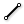Line (Ln)
Curve  >  Primitive  >  Line
Create a line between two points.
Inputs
Start Point (A)Line start point
End Point (B)Line end point
Outputs
Line (L)Line segment
and setting the start and end point.

An alternative is to use Line SDL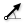Line SDL (Line)
Curve  >  Primitive  >  Line SDL
Create a line segment defined by start point, tangent and length.}
Inputs
Start (S)Line start point
Direction (D)Line tangent (direction)
Length (L)Line length
Outputs
Line (L)Line segment
, which takes the start point S, the direction vector D and the length L as input.

A line can also be created as a line of best fit for a collection of points, using the component Fit Line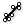Fit Line (FLine)
Curve  >  Primitive  >  Fit Line
Fit a line to a collection of points.
Inputs
Points (P)Points to fit
Outputs
Line (L)Line segment
.

### Convert curves to lines

If we have a curve that we need to convert to a line, we can extract the End PointsEnd Points (End)
Curve  >  Analysis  >  End Points
Extract the end points of a curve.
Inputs
Curve (C)Curve to evaluate
Outputs
Start (S)Curve start point
End (E)Curve end point
of the curve and use them to create a LineLine (Ln)
Curve  >  Primitive  >  Line
Create a line between two points.
Inputs
Start Point (A)Line start point
End Point (B)Line end point
Outputs
Line (L)Line segment
. Depending on the curve, a single line might be a bad approximation. For these cases, we should convert the curve to a polyline and then convert the line segments to lines.

### Convert polylines to lines

We can use Explode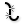Explode (Explode)
Curve  >  Util  >  Explode
Explode a curve into smaller segments.
Inputs
Curve (C)Curve to explode
Recursive (R)Recursive decomposition until all segments are atomic
Outputs
Segments (S)Exploded segments that make up the base curve
Vertices (V)Vertices of the exploded segments
to split a polyline into its individual segments. This component will also output the vertices of the polyline.

### Convert closed curve to lines

To convert a closed curve, like a circle, to lines, we first have to create a set of points on this curve and then we can use Shift List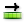Shift List (Shift)
Sets  >  List  >  Shift List
Offset all items in a list.
Inputs
List (L)List to shift
Shift (S)Shift offset
Wrap (W)Wrap values
Outputs
List (L)Shifted list
to offset the list indices of end points. Then we use LineLine (Ln)
Curve  >  Primitive  >  Line
Create a line between two points.
Inputs
Start Point (A)Line start point
End Point (B)Line end point
Outputs
Line (L)Line segment
to create the lines.

An alternative is using the points as vertices for a PolyLine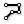PolyLine (PLine)
Curve  >  Spline  >  PolyLine
Create a polyline connecting a number of points.
Inputs
Vertices (V)Polyline vertex points
Closed (C)Close polyline
Outputs
Polyline (Pl)Resulting polyline
and we get the individual lines with ExplodeExplode (Explode)
Curve  >  Util  >  Explode
Explode a curve into smaller segments.
Inputs
Curve (C)Curve to explode
Recursive (R)Recursive decomposition until all segments are atomic
Outputs
Segments (S)Exploded segments that make up the base curve
Vertices (V)Vertices of the exploded segments
. We have to set input C of PolyLine to True to get a closed polyline.

### Convert grid points to lines

If we have a grid, or any ordered list of points, there are two methods to create lines between the points. The first one is to create a PolyLinePolyLine (PLine)
Curve  >  Spline  >  PolyLine
Create a polyline connecting a number of points.
Inputs
Vertices (V)Polyline vertex points
Closed (C)Close polyline
Outputs
Polyline (Pl)Resulting polyline
with the list points as vertices and then use ExplodeExplode (Explode)
Curve  >  Util  >  Explode
Explode a curve into smaller segments.
Inputs
Curve (C)Curve to explode
Recursive (R)Recursive decomposition until all segments are atomic
Outputs
Segments (S)Exploded segments that make up the base curve
Vertices (V)Vertices of the exploded segments
to extract the lines.

The second method uses the LineLine (Ln)
Curve  >  Primitive  >  Line
Create a line between two points.
Inputs
Start Point (A)Line start point
End Point (B)Line end point
Outputs
Line (L)Line segment
component: As start points we need every point except the last one and as end points we need all but the first one. We can use Cull Index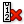Cull Index (Cull i)
Sets  >  Sequence  >  Cull Index
Cull (remove) indexed elements from a list.
Inputs
List (L)List to cull
Indices (I)Culling indices
Wrap (W)Wrap indices to list range
Outputs
List (L)Culled list
with index I set to -1 to remove the last item and Cull IndexCull Index (Cull i)
Sets  >  Sequence  >  Cull Index
Cull (remove) indexed elements from a list.
Inputs
List (L)List to cull
Indices (I)Culling indices
Wrap (W)Wrap indices to list range
Outputs
List (L)Culled list
with input I set to 0 to remove the first item.Flip Matrix is used to get the lines in the other direction.

## Polylines

### Construct polylines

The component PolyLinePolyLine (PLine)
Curve  >  Spline  >  PolyLine
Create a polyline connecting a number of points.
Inputs
Vertices (V)Polyline vertex points
Closed (C)Close polyline
Outputs
Polyline (Pl)Resulting polyline
creates a polyline with the provided vertices V. The polygonal chain runs in the order that the provided list of points has. At input C we can toggle whether the last and first point are connected to create a closed curve. This is False by default.

If we have a collection of lines, we can use Join Curves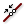Join Curves (Join)
Curve  >  Util  >  Join Curves
Join as many curves as possible
Inputs
Curves (C)Curves to join
Preserve (P)Preserve direction of input curves
Outputs
Curves (C)Joined curves and individual curves that could not be joined.
to combine them to segments in a polyline.

Also, every polygonal shape will automatically be converted to a polyline at a component’s input, which is requiring a curve.

### Deconstruct polylines

To deconstruct a polyline, there are two components that can be used. ExplodeExplode (Explode)
Curve  >  Util  >  Explode
Explode a curve into smaller segments.
Inputs
Curve (C)Curve to explode
Recursive (R)Recursive decomposition until all segments are atomic
Outputs
Segments (S)Exploded segments that make up the base curve
Vertices (V)Vertices of the exploded segments
splits the polyline at its vertices and outputs the segments S and the vertices V. The component Discontinuity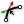Discontinuity (Disc)
Curve  >  Analysis  >  Discontinuity
Find all discontinuities along a curve.
Inputs
Curve (C)Curve to analyze
Level (L)Level of discontinuity to test for (1=C1, 2=C2, 3=Cinfinite)
Outputs
Points (P)Points at discontinuities
Parameters (t)Curve parameters at discontinuities
splits a polyline at the kinks and outputs the vertices P and the curve parameters t.
There can be fewer kinks than vertices in a polyline. See the section about rebuilding polylines below to see how this can be used as an advantage.
There can be fewer kinks than vertices in a polyline. See the section about rebuilding polylines below to see how this can be used as an advantage.

### Convert curves to polylines

To convert a curve to a polyline, we can use Curve To Polyline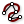Curve To Polyline (ToPoly)
Curve  >  Util  >  Curve To Polyline
Convert a curve to a polyline.
Inputs
Curve (C)Curve to simplify
Tolerance (distance) (Td)Deviation tolerance
Tolerance (angle) (Ta)Angle tolerance in radians
MinEdge (E-)Optional minimum allowed segment length
MaxEdge (E+)Optional maximum allowed segment length
Outputs
Polyline (P)Converted curve
Segments (S)Number of polyline segments
. We can set different parameters for the approximation: deviation tolerance Td and angle tolerance Ta and also minimum E- and maximum E+ allowed segment length.

We can also divide a curve into segments of equal length with Divide Curve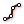Divide Curve (Divide)
Curve  >  Division  >  Divide Curve
Divide a curve into equal length segments
Inputs
Curve (C)Curve to divide
Count (N)Number of segments
Kinks (K)Split segments at kinks
Outputs
Points (P)Division points
Tangents (T)Tangent vectors at division points
Parameters (t)Parameter values at division points
. Afterward, we create a PolyLinePolyLine (PLine)
Curve  >  Spline  >  PolyLine
Create a polyline connecting a number of points.
Inputs
Vertices (V)Polyline vertex points
Closed (C)Close polyline
Outputs
Polyline (Pl)Resulting polyline
from the resulting points. This method should only be used if we need the equal length feature, for example to subdivide lines for FEM analysis. In general, Curve To PolylineCurve To Polyline (ToPoly)
Curve  >  Util  >  Curve To Polyline
Convert a curve to a polyline.
Inputs
Curve (C)Curve to simplify
Tolerance (distance) (Td)Deviation tolerance
Tolerance (angle) (Ta)Angle tolerance in radians
MinEdge (E-)Optional minimum allowed segment length
MaxEdge (E+)Optional maximum allowed segment length
Outputs
Polyline (P)Converted curve
Segments (S)Number of polyline segments
is the better approach.Input C was set to True to generate a closed curve for the approximation of the circle.

### Rebuild polylines

In this section, we look at two approaches to rebuild or simplify a polyline based on its general characteristic. The first one is to remove some points while maintaining most of the polyline’s characteristics, the second approach is to create some kind of fitting polyline, thus reducing some of the peaks. If you would like to add or remove specific vertices or tweak a polyline at a certain point, you find the answers in the how-to Modify polylines.

First, we take a look at a discreet modification of the polyline: If there are segments that have the same direction vector as the previous one, thus there is no kink at a vertex, we can remove this vertex without changing the characteristic of the polyline. To illustrate this, we use a polyline with two legs, but one leg has a point P in the middle, which can be set to deviate from its initial position to P' (see figure). Thus, the initial polyline consists of three segments; but visually, only two are recognizable.

With no kink, we have three options: The combination of DiscontinuityDiscontinuity (Disc)
Curve  >  Analysis  >  Discontinuity
Find all discontinuities along a curve.
Inputs
Curve (C)Curve to analyze
Level (L)Level of discontinuity to test for (1=C1, 2=C2, 3=Cinfinite)
Outputs
Points (P)Points at discontinuities
Parameters (t)Curve parameters at discontinuities
and PolyLinePolyLine (PLine)
Curve  >  Spline  >  PolyLine
Create a polyline connecting a number of points.
Inputs
Vertices (V)Polyline vertex points
Closed (C)Close polyline
Outputs
Polyline (Pl)Resulting polyline
, Reduce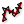Reduce (RedPLine)
Curve  >  Util  >  Reduce
Reduce a polyline by removing least significant vertices.
Inputs
Polyline (P)Polyline to reduce
Tolerance (T)Tolerance (allowed deviation between original and reduction)
Outputs
Polyline (P)Reduced polyline
Reduction (R)Number of vertices removed during reduction
and Simplify Curve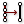Simplify Curve (Simplify)
Curve  >  Util  >  Simplify Curve
Simplify a curve.
Inputs
Curve (C)Curve to simplify
Tolerance (t)Optional deviation tolerance (if omitted, the current document tolerance is used)
Angle Tolerance (a)Optional angle tolerance (if omitted, the current document tolerance is used)
Outputs
Curve (C)Simplified curve
Simplified (S)True if curve was modified in any way
. They all return a new polyline with 2 instead of 3 segments.Removing a vertex when P = P'

Now, we move the point P by 0.5 to position P'. Also, we assign 0.5 as tolerance T to ReduceReduce (RedPLine)
Curve  >  Util  >  Reduce
Reduce a polyline by removing least significant vertices.
Inputs
Polyline (P)Polyline to reduce
Tolerance (T)Tolerance (allowed deviation between original and reduction)
Outputs
Polyline (P)Reduced polyline
Reduction (R)Number of vertices removed during reduction
and 1 as tolerance t and as angle a to Simplify CurveSimplify Curve (Simplify)
Curve  >  Util  >  Simplify Curve
Simplify a curve.
Inputs
Curve (C)Curve to simplify
Tolerance (t)Optional deviation tolerance (if omitted, the current document tolerance is used)
Angle Tolerance (a)Optional angle tolerance (if omitted, the current document tolerance is used)
Outputs
Curve (C)Simplified curve
Simplified (S)True if curve was modified in any way
. We can see that both components rebuild the curve by jumping over the deviating point. Thus, we get 2 segments instead of 3. The set tolerance is actually the same at both components and is best illustrated by reference to a circle: input T at Reduce resembles a radius while t at Simplify Curve resembles the diameter.

How do both components differ? Simplify Curve will handle all kinds of curves and we can set a deviation angle. Reduce will, if the polyline has a high amplitude and short frequency, reduce vertices and so the oscillation, while keeping the peak values. The component calls it the reduction of the least significant points and this could be useful for a dataset of measurements.Removing a vertex when P ≠ P'

Another component to reduce the number of segments is Polyline Collapse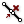Polyline Collapse (PCol)
Curve  >  Util  >  Polyline Collapse
Collapse short segments in a polyline curve.
Inputs
Polyline (P)Polyline curve
Tolerance (t)Segment length tolerance
Outputs
Polyline (Pl)Resulting polyline
Count (N)Number of segments that were collapsed
. As visualized in the figure, we continue with the setup, but point P is moved towards point Q. At Polyline Collapse, we set a segment length tolerance t of 2. The component will now remove segments that are shorter than the tolerance and create a new vertex V' at the midpoint of the removed segment. The length and direction of the adjacent segments will change accordingly.

This kind of operation can be useful, when lots of short lines are imported from another source, for example an area map. We can use Join CurvesJoin Curves (Join)
Curve  >  Util  >  Join Curves
Join as many curves as possible
Inputs
Curves (C)Curves to join
Preserve (P)Preserve direction of input curves
Outputs
Curves (C)Joined curves and individual curves that could not be joined.
to combine the lines to segments of a polyline and then generate a polyline with fewer vertices.Removing a segment with Polyline Collapse

To address the second approach, creating some kind of fitting curve with the initial vertices, we can use the component Smooth Polyline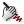Smooth Polyline (SmoothPLine)
Curve  >  Util  >  Smooth Polyline
Smooth the vertices of a polyline curve.
Inputs
Polyline (P)Polyline to smooth
Strength (S)Smoothing strength (0 = none, 1 = maximum)
Times (T)Number of times to apply the smoothing operation
Outputs
Polyline (P)Smoothed polyline
. Though, the components above can be used (when provided with large tolerances) to radically change the polyline, it is not what they were meant to do. Smooth Polyline rebuilds the polyline by reducing the height of the peaks; the polyline’s oscillation. We can set the strength of the smoothing S and the number of times T this function is applied. After several iterations with high strength, the polyline will lose its oscillation and become a line, stretching from its initial start to end point.Creating a fitting curve with the initial vertices.
This page is open source. Edit it on GitHub or see how you can contribute.